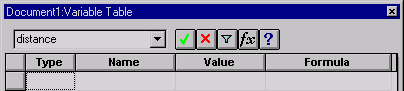# Using the Variable Table - SmartSketch - Help

## SmartSketch Help

PPMProduct
SmartSketch
PPMCategory_custom
Help
Version_SPEM_custom
9.0.0
Version_SSK
9.0(2015)

You can use the Variable Table to define and edit functional relationships between the dimensions of a design in a familiar spreadsheet format. The Variable Table is accessed with Variables on the Tools menu. There are two types of variables: dimensions displayed in the design and variables you create directly in the Variable Table. Dimension variables directly control elements of a design. The variable you assign must be set equal to a value or mathematical expression; for example, PI = 3.14159.

You can use variables to do the following:

• Drive a dimension with another dimension; Dimension A = Dimension B

• Define a constant; pi=3.14

• Drive a dimension with a formula; Dimension A = pi * 3.5

• Drive a dimension with a formula and another dimension; Dimension A = pi * Dimension B

• Drive a dimension with a formula that includes a function; Dimension A = Dimension B + cos (Dimension C)

• Drive a dimension with a value from a spreadsheet, such as a Microsoft Excel document, by copying the value from the spreadsheet into the Variable Table. Besides Excel, you can use any other spreadsheet software that can link or embed objects.

Accessing the Variable Table

After you select Variables on the Tools menu, a table appears with the following columns: Type, Name, Value, and Formula. Each row of the table displays a variable.Entering Data into the Variable Table

When you open the Variable Table in the document, all the dimensional values for the dimensions on the drawing sheet appear in the columns. The name that appears in the Name column is automatically generated by the software. You can change the name to something more logical if you want.

Restricting the Display of Variables

You can restrict the display of variables in the table using Filter on the Variable Table window:

• You can display only the variables for dimensions that the software created.

• You can also display variables that are associated with elements in the current document or the active window.

• You can also display a set of elements that you have selected in the document.

Creating Expressions

The system provides a set of standard mathematical functions. The functions can be typed in with the proper syntax or you can use the Function Wizard to select and define the function. The Function Wizard is convenient when you forget the proper syntax for a math function. You start the Function Wizard by clicking Fx in the Variable Table. The function is written in the Formula column of the selected row.

Examples

Suppose you draw a sheet metal bracket and you want to build a relationship between the bend radius and stock thickness. You can use a formula in the Variable Table to build and manage this relationship. The following example illustrates how the Variable Table would look if you built a relationship that changes the bend radius when the stock thickness changes.

Type

Name

Value

Formula

Variable

Stock_thickness

.25

Dimension

.375

1.5 * stock_thickness

Here are some more examples of how you might set up the Variable Table:

Type

Name

Value

Formula

Variable

c

2.0 kg

Variable

d

@c:\bearing.xls!sheet1!R6C3

Variable

e

20 mm

@c:\bearing.xls!sheet1R!6C3

Argument Conventions

The following argument conventions are used in the Variable Table:

• In the syntax line, required arguments are bold and optional arguments are not.

• Argument names should follow the rules for Visual Basic.

• In the text where functions and arguments are defined, required and optional arguments are not bold. Use the format in the syntax line to determine whether an argument is required or optional.